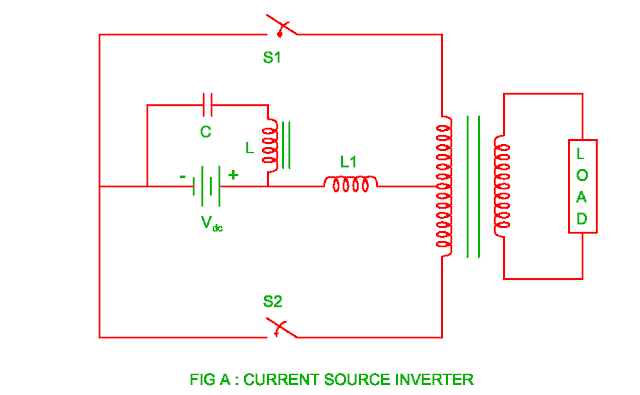## 22/05/2017

### Current Source Inverter ( CSI )

• The voltage source inverter is one in which output voltage remains constant and it does not depends upon type of load.
• The voltage across load is kept constant by connecting large capacitor at the input side of the inverter.
• The current source inverter is one in which output current remains constant with respect to type of load.
• An inductor of suitable value is placed in series with DC voltage source.• The DC input voltage in the current source inverter is supplied through rectifier bridge/controlled rectifier bridge or DC chopper.
• The LC filter is connected at the input in order to get ripple free current.
• The function of the inductor L is to decrease the ripple current whereas the capacitor C is to reduce source impedance.
• The power flow from supply to load and vice versa is possible at different power factor due to low impedance of DC supply.
• The current source inverter is either load commutated or force commutated
• The current source inverter is load commutated if the load power factor is leading whereas it must be force commutated if the load power factor is lagging.
• The current source inverter can be used for the speed control of induction motor in which variation of load torque occurs.
• There is additional diode requires for feeding the reactive power back to the supply when inductive load is used.